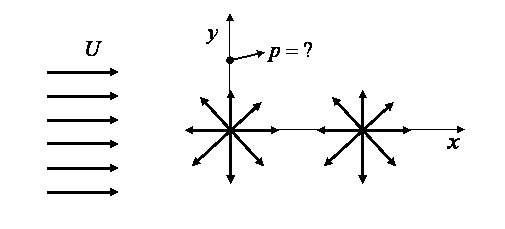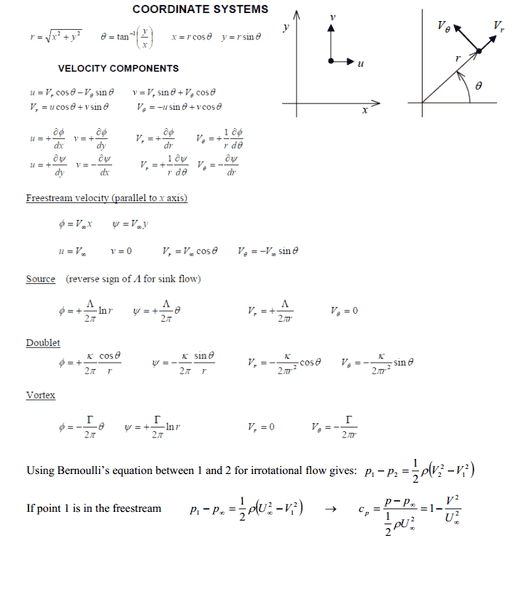# Fluids: potential flow, calculating gauge pressure from two sources

## Homework Statement

In a plane onset flow of 4 m/s, a source of strength 13 m2/s per metre is located at the origin (x=0, y=0) and another source of strength 18 m2/s per metre is located at (x=3m, y=0). Calculate the gauge pressure at the point (0, 2m), Take ρ =1.2kg/m3## Homework Equations## The Attempt at a Solution

I have attempted to work out the velocities, 13/(2pi*√x^2+y^2) and 18/(2pi*√(x-3)^2+y^2) and θ = 1.249 radians, although am somewhat unsure of how to proceed.

any help would be greatly appreciated! thanks

## Answers and Replies

Chestermiller
Mentor
What is the magnitude of the velocity vector associated with each of the two sources at the point p?

What are the components of these velocity vectors in the x- and y-directions at point p?

What are the components of the velocity vector associated with the flow U at point p?

What are the components of the overall velocity vector at point p?

What are the components of the velocity of the fluid at infinity?

I presume that you need to calculate the gauge pressure as the pressure at point p minus the pressure at infinity.